## Cissoid of DioclesA curve invented by Diocles in about 180 BCin connection with his attempt to duplicate the cube by geometrical methods. The name cissoid'' first appears in the work of Geminus about 100 years later. Fermatand Roberval constructed the tangent in 1634. Huygensand Wallis found, in 1658, that the Area between the curve and its asymptote was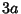(MacTutor Archive). From a given point there are either one or three Tangents to the cissoid.

Given an origin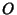and a pointon the curve, letbe the point where the extension of the lineintersects the line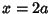andbe the intersection of the Circle of Radiusand centerwith the extension of. Then the cissoid of Diocles is the curve which satisfies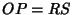.

The cissoid of Diocles is the Roulette of the Vertex of a Parabola rolling on an equal Parabola. Newtongave a method of drawing the cissoid of Diocles using two line segments of equal length at Right Angles. If they are moved so that one line always passes through a fixed point and the end of the other line segment slides along a straight line, then the Midpoint of the sliding line segment traces out a cissoid of Diocles.

The cissoid of Diocles is given by the parametric equations(1)(2)

Converting these to Polar Coordinates gives(3)

so(4)

In Cartesian Coordinates,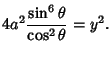(5)

An equivalent form is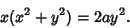(6)

Using the alternative parametric form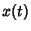(7)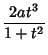(8)

(Gray 1993), gives the Curvature as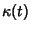(9)

References

Gray, A. The Cissoid of Diocles.'' §3.4 in Modern Differential Geometry of Curves and Surfaces.Boca Raton, FL: CRC Press, pp. 43-46, 1993.

Lawrence, J. D. A Catalog of Special Plane Curves. New York: Dover, pp. 98-100, 1972.

Lee, X. Cissoid of Diocles.'' http://www.best.com/~xah/SpecialPlaneCurves_dir/CissoidOfDiocles_dir/cissoidOfDiocles.html.

Lockwood, E. H. A Book of Curves. Cambridge, England: Cambridge University Press, pp. 130-133, 1967.

MacTutor History of Mathematics Archive. Cissoid of Diocles.'' http://www-groups.dcs.st-and.ac.uk/~history/Curves/Cissoid.html.

Yates, R. C. Cissoid.'' A Handbook on Curves and Their Properties. Ann Arbor, MI: J. W. Edwards, pp. 26-30, 1952.